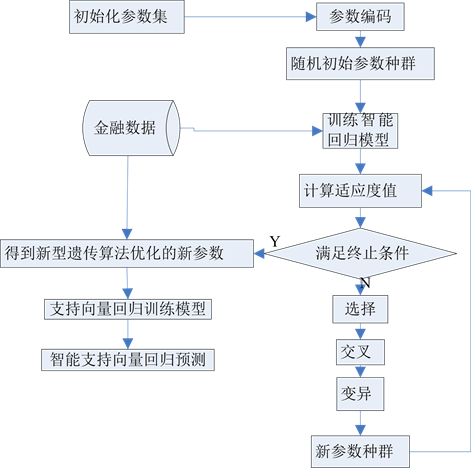# 基于智能支持向量机回归模型的金融数据预测Prediction of Finance Data Based Intelligent Support Vector Regression

DOI: 10.12677/CSA.2018.86105, PDF, HTML, XML, 下载: 679  浏览: 1,343

Abstract: Aiming at nonlinear, time variant, random, fuzziness and uncertainty of finance data, we propose a new intelligent support vector regression model and use new genetic algorithm to optimize the model’s parameters. Experiment results show that intelligent support vector regression has higher accuracy and runs faster than BP Neural Networks.

1. 引言

2. 支持向量回归模型

$g\left(x\right)=w\varphi \left(x\right)+b$ (1)

$\begin{array}{l}\mathrm{min}\text{\hspace{0.17em}}\text{\hspace{0.17em}}\text{\hspace{0.17em}}\frac{1}{2}{‖w‖}_{1}{}^{2}+C\underset{i=1}{\overset{n}{\sum }}\left({\xi }_{i}+{\xi }_{i}^{*}\right)\\ \text{s}\text{.t}\text{.}\text{\hspace{0.17em}}\text{\hspace{0.17em}}\text{\hspace{0.17em}}\text{\hspace{0.17em}}\text{ }{y}_{i}-w\varphi \left({x}_{i}\right)-b\le \epsilon +{\xi }_{i}\\ \text{\hspace{0.17em}}\text{\hspace{0.17em}}\text{\hspace{0.17em}}\text{\hspace{0.17em}}\text{\hspace{0.17em}}\text{\hspace{0.17em}}\text{\hspace{0.17em}}\text{\hspace{0.17em}}w\varphi \left({x}_{i}\right)+b-{y}_{i}\le \epsilon +{\xi }_{i}^{*},{\xi }^{\left(*\right)}\ge 0\end{array}$ (2)

$W\left({\alpha }_{i},{\alpha }_{i}^{*}\right)=\underset{i=1}{\overset{n}{\sum }}{y}_{i}\left({\alpha }_{i}-{\alpha }_{i}^{*}\right)-\epsilon \underset{i=1}{\overset{n}{\sum }}\left({\alpha }_{i}+{\alpha }_{i}^{*}\right)-\frac{1}{2}\underset{i=1}{\overset{n}{\sum }}\underset{i=1}{\overset{n}{\sum }}\left({\alpha }_{i}-{\alpha }_{i}^{*}\right)\left({\alpha }_{j}-{\alpha }_{j}^{*}\right)\left(\varphi \left({x}_{i}\right)\cdot \varphi \left({x}_{j}\right)\right)$ (3)

$g\left(x\right)=\underset{i=1}{\overset{n}{\sum }}\left({\alpha }_{i}-{\alpha }_{i}^{*}\right)\varphi \left({x}_{i}\right)\cdot \varphi \left(x\right)+b$ (4)

$g\left(x\right)=\underset{i=1}{\overset{n}{\sum }}\left({\alpha }_{i}-{\alpha }_{i}^{*}\right)K\left({x}_{i},x\right)+b$ (5)

$L\left(g\left({x}_{i}\right),{y}_{i}\right)=\left\{\begin{array}{l}|g\left({x}_{i}\right)-{y}_{i}|-\epsilon ,\text{\hspace{0.17em}}\text{\hspace{0.17em}}|g\left({x}_{i}\right)-{y}_{i}|\ge \epsilon \\ 0,\text{\hspace{0.17em}}\text{\hspace{0.17em}}\text{\hspace{0.17em}}\text{\hspace{0.17em}}\text{\hspace{0.17em}}\text{\hspace{0.17em}}\text{\hspace{0.17em}}\text{\hspace{0.17em}}\text{\hspace{0.17em}}\text{\hspace{0.17em}}\text{\hspace{0.17em}}\text{\hspace{0.17em}}\text{\hspace{0.17em}}\text{\hspace{0.17em}}\text{\hspace{0.17em}}\text{\hspace{0.17em}}\text{\hspace{0.17em}}\text{\hspace{0.17em}}\text{\hspace{0.17em}}\text{\hspace{0.17em}}\text{\hspace{0.17em}}\text{\hspace{0.17em}}\text{\hspace{0.17em}}\text{others}\end{array}$ (6)

3. 新型遗传算法

3.1. 适应度函数

$g=-\stackrel{¯}{MAP{E}_{CV}}$ (7)

$MAP{E}_{CV}=\frac{\underset{i=1}{\overset{n}{\sum }}\frac{|{y}_{i}-{g}_{i}|}{2{y}_{i}}}{n}×100%$ (8)

3.2. 编码方式

3.3. 选择操作

${P}_{i}=c{\left(1-3c\right)}^{i-1}$ (9)

3.4. 交叉和变异操作

${P}_{c}=\left\{\begin{array}{l}{P}_{{c}_{1}}-\left({P}_{{c}_{1}}-{P}_{{c}_{2}}\right)\left({g}^{\prime }-{g}_{avg}\right)/\left({g}_{\mathrm{max}}-{g}_{avg}\right),\text{\hspace{0.17em}}\text{\hspace{0.17em}}{g}^{\prime }\ge {g}_{avg}\\ {P}_{{c}_{1}},\text{\hspace{0.17em}}\text{\hspace{0.17em}}\text{\hspace{0.17em}}\text{\hspace{0.17em}}\text{\hspace{0.17em}}\text{\hspace{0.17em}}\text{\hspace{0.17em}}\text{\hspace{0.17em}}\text{\hspace{0.17em}}\text{\hspace{0.17em}}\text{\hspace{0.17em}}\text{\hspace{0.17em}}\text{\hspace{0.17em}}\text{\hspace{0.17em}}\text{\hspace{0.17em}}\text{\hspace{0.17em}}\text{\hspace{0.17em}}\text{\hspace{0.17em}}\text{\hspace{0.17em}}\text{\hspace{0.17em}}\text{\hspace{0.17em}}\text{\hspace{0.17em}}\text{\hspace{0.17em}}\text{\hspace{0.17em}}\text{\hspace{0.17em}}\text{\hspace{0.17em}}\text{\hspace{0.17em}}\text{\hspace{0.17em}}\text{\hspace{0.17em}}\text{\hspace{0.17em}}\text{\hspace{0.17em}}\text{\hspace{0.17em}}\text{\hspace{0.17em}}\text{\hspace{0.17em}}\text{\hspace{0.17em}}\text{\hspace{0.17em}}\text{\hspace{0.17em}}\text{\hspace{0.17em}}\text{\hspace{0.17em}}\text{\hspace{0.17em}}\text{\hspace{0.17em}}\text{\hspace{0.17em}}\text{\hspace{0.17em}}\text{\hspace{0.17em}}\text{\hspace{0.17em}}\text{\hspace{0.17em}}\text{\hspace{0.17em}}\text{\hspace{0.17em}}\text{\hspace{0.17em}}\text{\hspace{0.17em}}\text{ }\text{\hspace{0.17em}}\text{\hspace{0.17em}}\text{\hspace{0.17em}}\text{\hspace{0.17em}}{g}^{\prime }<{g}_{avg}\end{array}$ (10)

${P}_{m}=\left\{\begin{array}{l}{P}_{{m}_{1}}-\left({P}_{{m}_{1}}-{P}_{{m}_{2}}\right)\left({f}_{\mathrm{max}}-g\right)/\left({g}_{\mathrm{max}}-{g}_{avg}\right),\text{\hspace{0.17em}}\text{\hspace{0.17em}}{g}^{\prime }\ge {g}_{avg}\\ {P}_{{m}_{1}},\text{\hspace{0.17em}}\text{\hspace{0.17em}}\text{\hspace{0.17em}}\text{\hspace{0.17em}}\text{\hspace{0.17em}}\text{\hspace{0.17em}}\text{\hspace{0.17em}}\text{\hspace{0.17em}}\text{\hspace{0.17em}}\text{\hspace{0.17em}}\text{\hspace{0.17em}}\text{\hspace{0.17em}}\text{\hspace{0.17em}}\text{\hspace{0.17em}}\text{\hspace{0.17em}}\text{\hspace{0.17em}}\text{\hspace{0.17em}}\text{\hspace{0.17em}}\text{\hspace{0.17em}}\text{\hspace{0.17em}}\text{\hspace{0.17em}}\text{\hspace{0.17em}}\text{\hspace{0.17em}}\text{\hspace{0.17em}}\text{\hspace{0.17em}}\text{\hspace{0.17em}}\text{\hspace{0.17em}}\text{\hspace{0.17em}}\text{\hspace{0.17em}}\text{\hspace{0.17em}}\text{\hspace{0.17em}}\text{\hspace{0.17em}}\text{\hspace{0.17em}}\text{\hspace{0.17em}}\text{\hspace{0.17em}}\text{\hspace{0.17em}}\text{\hspace{0.17em}}\text{\hspace{0.17em}}\text{\hspace{0.17em}}\text{\hspace{0.17em}}\text{\hspace{0.17em}}\text{\hspace{0.17em}}\text{\hspace{0.17em}}\text{\hspace{0.17em}}\text{\hspace{0.17em}}\text{\hspace{0.17em}}\text{\hspace{0.17em}}\text{\hspace{0.17em}}\text{\hspace{0.17em}}\text{\hspace{0.17em}}\text{ }\text{\hspace{0.17em}}\text{\hspace{0.17em}}\text{ }\text{ }\text{\hspace{0.17em}}\text{\hspace{0.17em}}{g}^{\prime }<{g}_{avg}\end{array}$ (11)

${P}_{c}^{t}=\left\{\begin{array}{l}{P}_{{c}_{1}}\cdot \sqrt{1-{\left(t/{t}_{\mathrm{max}}\right)}^{2}},\text{\hspace{0.17em}}\text{\hspace{0.17em}}\text{\hspace{0.17em}}{P}_{c}^{t}<{P}_{{c}_{2}},\\ {P}_{{c}_{2}},\text{\hspace{0.17em}}\text{\hspace{0.17em}}\text{\hspace{0.17em}}\text{\hspace{0.17em}}\text{\hspace{0.17em}}\text{\hspace{0.17em}}\text{\hspace{0.17em}}\text{\hspace{0.17em}}\text{\hspace{0.17em}}\text{\hspace{0.17em}}\text{\hspace{0.17em}}\text{\hspace{0.17em}}\text{\hspace{0.17em}}\text{\hspace{0.17em}}\text{\hspace{0.17em}}\text{\hspace{0.17em}}\text{\hspace{0.17em}}\text{\hspace{0.17em}}\text{\hspace{0.17em}}\text{\hspace{0.17em}}\text{\hspace{0.17em}}\text{\hspace{0.17em}}\text{ }\text{\hspace{0.17em}}{P}_{c}^{t}\ge {P}_{{c}_{2}}\end{array}$ (12)

${P}_{m}^{t}=\left\{\begin{array}{l}{P}_{{m}_{1}}\cdot \mathrm{exp}\left(-\lambda \cdot t/{t}_{\mathrm{max}}\right),\text{\hspace{0.17em}}\text{\hspace{0.17em}}{P}_{m}^{t}>{P}_{{m}_{2}},\\ {P}_{{m}_{2}},\text{\hspace{0.17em}}\text{\hspace{0.17em}}\text{\hspace{0.17em}}\text{\hspace{0.17em}}\text{\hspace{0.17em}}\text{\hspace{0.17em}}\text{\hspace{0.17em}}\text{\hspace{0.17em}}\text{\hspace{0.17em}}\text{\hspace{0.17em}}\text{\hspace{0.17em}}\text{\hspace{0.17em}}\text{\hspace{0.17em}}\text{\hspace{0.17em}}\text{\hspace{0.17em}}\text{\hspace{0.17em}}\text{\hspace{0.17em}}\text{\hspace{0.17em}}\text{\hspace{0.17em}}\text{\hspace{0.17em}}\text{\hspace{0.17em}}\text{\hspace{0.17em}}\text{\hspace{0.17em}}\text{\hspace{0.17em}}\text{\hspace{0.17em}}\text{ }\text{ }{P}_{m}^{t}\le {P}_{{m}_{2}}\end{array}$ (13)

4. 智能支持向量回归模型模型及应用

4.1. 智能支持向量回归模型流程图

4.2. 数据收集

4.3. 数据标准化处理

4.4. 实验结果Figure 1. Flow chart of intelligent support vector regression modelTable 1. The comparison of intelligent support vector regression model

5. 结论

  盛丹姝, 王德辉, 刘书丽. 基于融合估计的金融数据预测[J]. 吉林师范大学学报(自然科学版), 2013(1): 38-41.  廖丽芳, 蔡如华. 基于MODWT在金融数据预测的应用[J].计算机工程与设计, 2013, 34(4): 1346-1350.  徐喆. 逻辑回归模型在互联网金融P2P业务信用风险的应用[J]. 统计科学与实践，2015(11): 26-29.  James, G., Witten, D., Hastie, T. and Tibshirani, R. (2013) An Introduction to Statistical Learning with Applications in R. Springer New York Heidlberg Dordrecht London. https://doi.org/10.1007/978-1-4614-7138-7  杨从锐, 钱谦, 王锋, 孙铭会. 改进的自适应遗传算法在函数优化中的应用[J]. 计算机应用研究, 2017, 35(4): 1-5.  Lin, C.D., Anderson-Cook, C.M., Hamada, M.S., et al. (2015) Using Genetic Algo-rithms to Design Experiments: A Review. Quality & Reliability Engineering International, 31, 155-167. https://doi.org/10.1002/qre.1591  Goren, H.G., Tunali, S. and Jans, R. (2010) A Review of Applications of Genetic Algo-rithms in Lot Sizing. Journal of Intelligent Manufacturing, 21, 575-590. https://doi.org/10.1007/s10845-008-0205-2